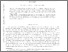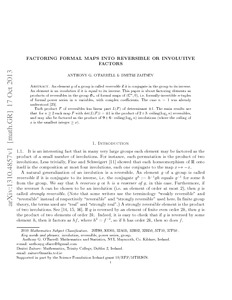# Factoring Formal Maps into Reversible or Involutive Factors

O'Farrell, Anthony G. and Zaitsev, Dmitri (2014) Factoring Formal Maps into Reversible or Involutive Factors. Journal of Algebra, 399. pp. 657-674. ISSN 0021-8693Previewmore...Add this article to your Mendeley library

## Abstract

An element g of a group is called reversible if it is conjugate in the group to its inverse. An element is an involution if it is equal to its inverse. This paper is about factoring elements as products of reversibles in the group Gn of formal maps of (Cn, 0), i.e. formally-invertible n-tuples of formal power series in n variables, with complex coefficients. The case n = 1 was already understood . Each product F of reversibles has linear part L(F) of determinant ±1. The main results are that for n ≥ 2 each map F with det(L(F)) = ±1 is the product of 2+3 • ceiling(log2 n) reversibles, and may also be factored as the product of 9 + 6 • ceiling(log2 n) involutions (where the ceiling of x is the smallest integer ≥ x).

Item Type: Article This is the preprint version of the published article, which is available at doi:10.1016/j.jalgebra.2013.09.053 Involution; Reversible; Power series; Group; Faculty of Science and Engineering > Mathematics and Statistics 6271 https://doi.org/10.1016/j.jalgebra.2013.09.053 Prof. Anthony O'Farrell 17 Jul 2015 14:51 Journal of Algebra Elsevier YesItem control page